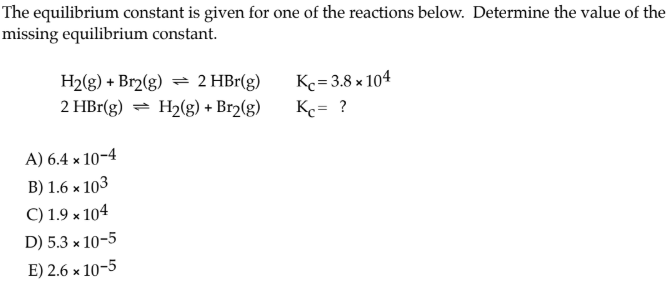# The equilibrium constant is given for one of the reactions below. Determine the value of the missing equilibrium constant. H2(g) + Br2(g) ⇌ 2 HBr(g) Kc = 3.8 × 10^4 2 HBr(g) ⇌ H2(g) + Br2(g) Kc = ? A) 6.4 × 10^-4 B) 1.6 × 10^3 C) 1.9 × 10^4 D) 5.3 × 10^-5 E) 2.6 × 10^-5